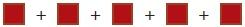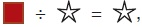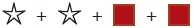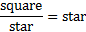# User ForumSubject :IMO    Class : Class 4

If= 125 andthen= ________.

A50
B25
C60
D45

it can be 50 also

## Ans 1: (Master Answer)

Class : Class 1

(C): Square + square+ square+ square+ square = 125

⇒ 5 × square = 125
⇒Square = 25
Now,⇒Star × star = 25
So, Star = 5
Hence, Star + star + square + square = 5 + 5 + 25 + 25 = 60